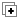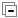## Excel Sheet Manipulation APIs

Excel sheets are a favourite among business users. Sahi has APIs to manipulate Excel Sheets which work on non-Windows machines too. Sahi uses Apache POI libraries underneath.

 Since: Sahi Pro Sahi OS Sahi Pro Starter Desktop Add-On Mobile Add-On SAP Add-On 4.0 NA NA 7.0.0 7.5.0 9.0.0

Available for modes: Browser | Windows | Java | Android | iOS | SAP

``_readExcelFile(\$filePath[, \$sheetName[, \$props]])``

Arguments
 \$filePath string path to Excel file. Can be of extension .xls or .xlsx \$sheetName string optional Sheet name. Defaults to sheetname of first sheet. \$props object optional Properties to specify for:ignoreEmptyRows: boolean: Ignores the empty lines. Defaults to false.includeHeader: boolean: If true, returns the header row also as the first row. Defaults to false.

Returns
 two dimensional array of strings a 2 dimensional array of all values in the excel file

Details

Reads the excel sheet and returns data as an associative array.

Given an Excel Sheet like

 A B C D E F G 1 Name Age 2 Ram 18 3 Amir 20 4
``var \$data = _readExcelFile("data.xls");``
\$data is now
``````[
{"Name":"Ram","Age":"18"}
{"Name":"Amir","Age":"20"}
]``````
It can be accessed like this:
``````_log("Name of first user is: " + \$data["Name"]); // logs "Ram"
_log("Age of second user is: " + \$data["Age"]); // logs "20"``````
\$props can be passed as following:
``````var \$data = _readExcelFile("data.xls");
var \$dataLength = \$data.length // returns 4 since includeHeader and ignoreEmptyRows default value is false
var \$data1Length = \$data1.length // returns 5 since includeHeader is true
var \$data2Length = \$data2.length // returns 4 since includeHeader and ignoreEmptyRows are true
var \$data3 = _readExcelFile("data.xls", null, {includeHeader:true,ignoreEmptyRows:true}); // In case of the default sheet name use 'null' or use sheet name there.``````

For more complex manipulation `_getExcel` can also be used. This allows modifying Excel sheets too.

### _getExcel

 Since: Sahi Pro Sahi OS Sahi Pro Starter Desktop Add-On Mobile Add-On SAP Add-On 4.0 NA NA 7.0.0 7.5.0 9.0.0

Available for modes: Browser | Windows | Java | Android | iOS | SAP

``_getExcel(\$filePath[, \$sheetName])``

Arguments
 \$filePath string path to Excel file. Can be of extension .xls or .xlsx \$sheetName string optional Sheet name. Defaults to sheetname of first sheet.

Returns
 object ExcelPOI object which exposes various APIs to manipulate the Excel

Details

The example below illustrates the usage of various APIs.
``````//Get a handle to the Excel sheet
var \$excel = _getExcel("D:/poiTest.xls","Sheet1");

//Set Data
var \$excelData=[["ajay","babu","cheeran"],["david","elango","frank"],
["gokul","hari","irfan"],["jo","kumar","latha"],["mani","naraen","ojha"],
["peter","queen","richard"],["shalini","thomas","umesh"]];
\$excel.setData(\$excelData);

//Get Data
var \$getdata=\$excel.getData();
_assertEqual("irfan",\$getdata);

//Insert New Row at end
var \$insertRowData=["kathir","femina","jandir"];
\$excel.insertRow(\$insertRowData);

//Insert New Row at given index
var \$insertRowData=["kathir","femina","jandir"];
\$excel.insertRow(\$insertRowData, 2); // will insert at index 2. Shifts other cells down

//Insert New Rows at end
var \$insertRowsData=[["shoba","ravi","vasi"],["kajol","rani","sharuk"]];
\$excel.insertRows(\$insertRowsData);

//Insert New Rows at given index
var \$insertRowsData=[["shoba","ravi","vasi"],["kajol","rani","sharuk"]];
\$excel.insertRows(\$insertRowsData, 2); // will insert at index 2. Shifts other cells down

//Delete Row at index 4
var \$deleteRowIndex=4;
\$excel.deleteRow(\$deleteRowIndex);

//Delete Rows indexed 1 and 4
var \$deleteRowsIndexes=[1,4];
\$excel.deleteRows(\$deleteRowsIndexes);

//Delete Rows from row 3 to end
\$excel.deleteRows(3);

//Delete Rows from row 3 to row 8
\$excel.deleteRows(3, 8);

//Get Cell Value at cell position 2,2
var \$excelGet=\$excel.get(2, 2);
_assertEqual("xezwanth", \$excelGet);

//Set Cell Value at cell position 2,2
var \$excelData=\$excel.set(2,2,"444-444-4444");

//Get String array of all Sheet Names on that Excel.
var \$arrayOfSheetNames = \$excel.getSheetNames();

//Get ExcelPoi Workbook object
var \$workbook = \$excel.getRawWorkbook();``````
info Since 6.0.0, the following changes have been done.
• If a cell has a formula, `getData` and `get` functions return value instead of formula. Added a method `getCellFormula` that returns formula.
• Added method `getSheetNames` that returns all the sheet names as a String array
• Added method `getRawWorkbook` which returns a Workbook POI object. This gives the user the flexibility to deal with the Workbook object directly
• Added `getCellObject` that returns a Cell POI object.
• Added `getCellFont` that returns a Font POI object.
Since 6.1.0, `\$excel.getDataForDataDrive()` API has been added which returns data in a format appropriate for passing into _dataDrive. See getDataForDataDrive sample usage.